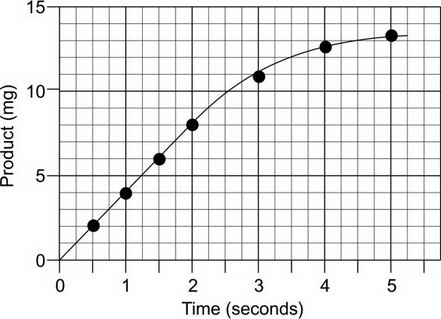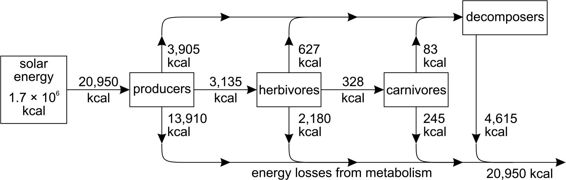# AP Biology Grid-Ins Practice Test 8

### Test Information6 questions9 minutes

1. A biochemist analyzed the composition of DNA extracted from cells of an unknown species. She found that the sample contained 28% adenine. What is the expected amount of cytosine? Express your answer as a percent to the nearest whole number.

2. A mutation of an X-linked, recessive allele is lethal. Male fetuses that inherit the allele and female fetuses that are homozygous recessive abort before birth. What is the probability that a child born to a female who is heterozygous for the mutation will have one copy of the allele? Express your answer as a decimal to the nearest hundredth.

3.The above graph shows the rate of an enzyme-mediated reaction. What is the rate of the reaction between 0 and 2 seconds? Express your answer as a decimal to the nearest tenth.

4.The figure above shows the flow of energy in a community. What percent of the energy taken in by producers ends up in carnivores? Express your answer as a percent to the nearest tenth.

5. A population in Hardy-Weinberg equilibrium consists of 300 sheep, some black and some white. If the allele frequency for the recessive white allele is 0.3, how many black sheep are there in the population?

6. The maximum rate of growth of a population is 0.5, and its carrying capacity is 2,000. What is the rate of population growth if the population size is 1,800?